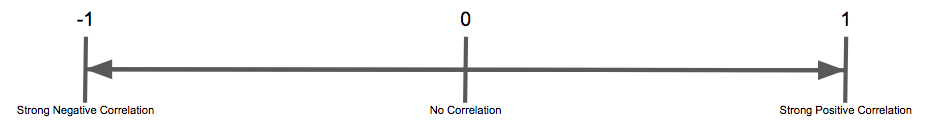# The Pearson Correlation Coefficient Formula in SQL

Posted by Tim Miller

## The Pearson Correlation Coefficient Formula in SQL

As we discussed on our “Using the PostgreSQL Correlation Function” page, using the correlation can show you how two series of numbers are related. Either their strength as a positive correlation or their strength as a negative correlation, and any amount of strength in between, including no correlation at all.

To recap a little bit on the Correlation Coefficient:

The Correlation Coefficient is a widely used method of determining the strength of the relationship between two numbers or two sets of numbers. This coefficient is calculated as a number between -1 and 1. 1 being the strongest possible positive correlation and -1 being the strongest possible negative correlation.A positive correlation means that as one number increases the second number will also increase.

A negative correlation means that as one number increases the second number decreases.

Whether or not the outcome of the second number is CAUSED by the first is not being determined here, just that the outcomes of the two numbers happen in concert with each other.

If the formula returns 0 then there is absolutely NO correlation between the two sets of numbers.

Pearson’s Formula looks like this:As was outlined, there are ways to calculate the Pearson Formula for a set of numbers in SQL.

We did it here on a number set from out demo data source, that comes free with a trial.

That formula in SQL looks like this:

``````((tot_sum - (amt_sum * act_sum / _count)) / sqrt((amt_sum_sq - pow(amt_sum, 2.0) / _count) * (act_sum_sq - pow(act_sum, 2.0) / _count)))
``````

And used in an entire query like this:

``````SELECT
((tot_sum - (amt_sum * act_sum / _count)) / sqrt((amt_sum_sq - pow(amt_sum, 2.0) / _count) * (act_sum_sq - pow(act_sum, 2.0) / _count))) AS "Corr Coef Using Pearson"

FROM(
SELECT
sum("Amount") AS amt_sum,
sum("Activities") AS act_sum,
sum("Amount" * "Amount") AS amt_sum_sq,
sum("Activities" * "Activities") AS act_sum_sq,
sum("Amount" * "Activities") AS tot_sum,
count(*) as _count

FROM(
SELECT
DATE_TRUNC('day', p.payment_date)::DATE AS "Day",
SUM(p.amount) AS "Amount",
COUNT(DISTINCT a.activity_id) AS "Activities"
FROM
public.payments p
INNER JOIN public.subscriptions s ON p.subscription_id = s.subscription_id
INNER JOIN public.users u ON s.user_id = u.user_id
INNER JOIN public.activity a ON a.user_id = u.user_id

GROUP BY 1) as a

) as b

GROUP BY tot_sum, amt_sum, act_sum, _count, amt_sum_sq, act_sum_sq
``````

What we would see from this query is exactly what we would see using the corr() function in PostgreSQL:#### similar articles## Introducing Visual SQL

SQL may be the language of data, but not everyone can understand it. With our visual version of SQL, now anyone at your company can query data from almost any source—no coding required.